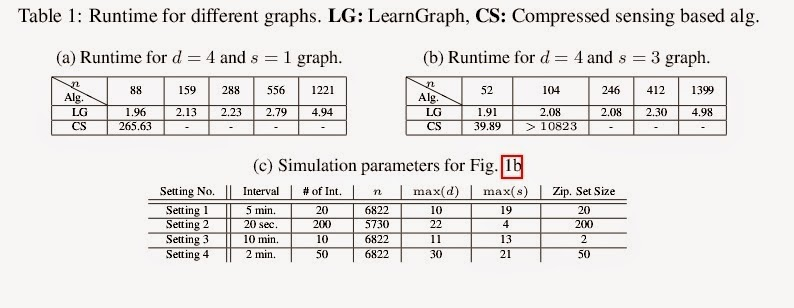## Tuesday, December 02, 2014

### Sparse Polynomial Learning and Graph Sketching

Very interesting connection between solving a CS problem  and sketching graph from random queries. I wonder it could help in devising biochemical networks.Sparse Polynomial Learning and Graph Sketching by Murat Kocaoglu, Karthikeyan Shanmugam,  Alexandros G. Dimakis, Adam Klivans

Let f: \{-1,1\}^n \rightarrow \mathbb{R} be a polynomial with at most s non-zero real coefficients. We give an algorithm for exactly reconstructing f given random examples from the uniform distribution on \{-1,1\}^n that runs in time polynomial in n and 2^{s} and succeeds if the function satisfies the \textit{unique sign property}: there is one output value which corresponds to a unique set of values of the participating parities. This sufficient condition is satisfied when every coefficient of f is perturbed by a small random noise, or satisfied with high probability when s parity functions are chosen randomly or when all the coefficients are positive. Learning sparse polynomials over the Boolean domain in time polynomial in n and 2^{s} is considered notoriously hard in the worst-case. Our result shows that the problem is tractable for almost all sparse polynomials. Then, we show an application of this result to hypergraph sketching which is the problem of learning a sparse (both in the number of hyperedges and the size of the hyperedges) hypergraph from uniformly drawn random cuts. We also provide experimental results on a real world dataset.
earlier: Learning Fourier Sparse Set Functions by Peter Stobbe Andreas Krause

Join the CompressiveSensing subreddit or the Google+ Community and post there !Liked this entry ? subscribe to Nuit Blanche's feed, there's more where that came from. You can also subscribe to Nuit Blanche by Email, explore the Big Picture in Compressive Sensing or the Matrix Factorization Jungle and join the conversations on compressive sensing, advanced matrix factorization and calibration issues on Linkedin.